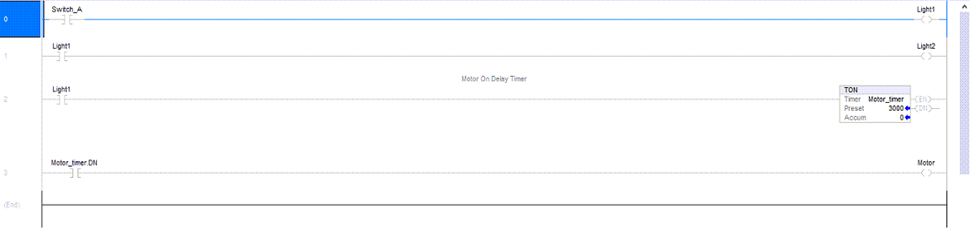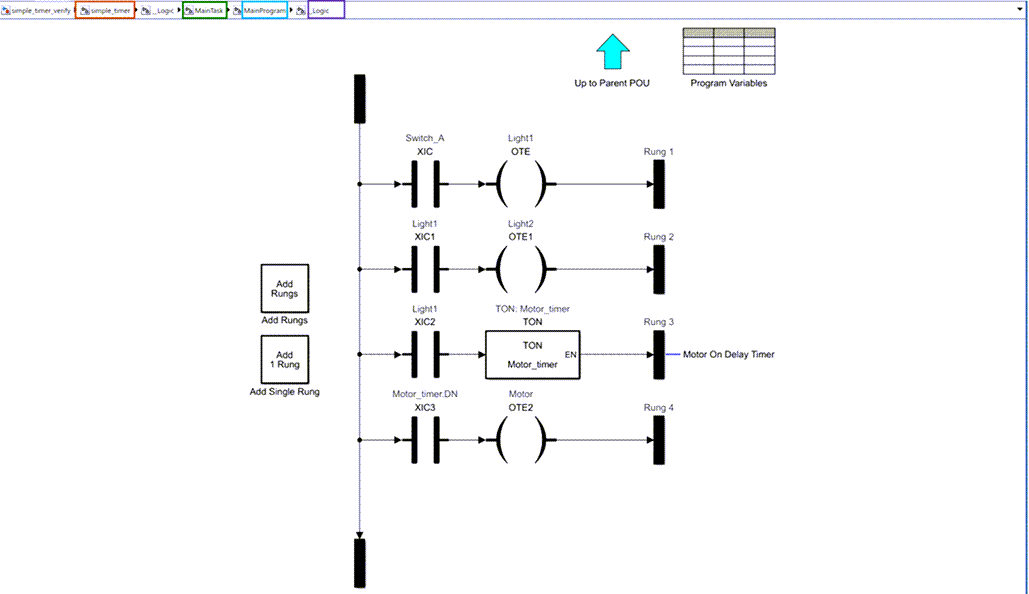Simulate, test, and validate your `.L5X `ladder diagram files by importing your ladder diagram files into Simulink®. Use the `plcimportladder `function to import your ladder diagram files into Simulink. Simulink PLC Coder™ supports only import of ladder diagram files created by using Rockwell Automation® RSLogix 5000® and Studio 5000 ® integrated development environments (IDEs).

The ladder diagram in the `simple_timer.L5X `file controls a motor by using an input switch (`Switch_A)` and a timer (`Motor_timer). `This ladder diagram was created using the Studio 5000 IDE.`Light1, Light2, and Motor `are the outputs of this ladder diagram.

Before using the `plcimportladder `function to import your ladder diagram files into Simulink:

• Verify that your .`L5X `ladder diagram` `file has no errors by compiling the file in the Rockwell Automation IDE.

• Verify that the `.L5X `ladder diagram file uses blocks that are supported by Simulink PLC Coder. For a list of supported blocks at the MATLAB® command line, enter:

```plcladderlib ```

To import the `simple_timer.L5X `ladder diagram file into Simulink, use the `plcimportladder `function.

`plcimportladder('simple_timer.L5X');`

The ladder diagram is imported into Simulink and a `simple_timer.slx `file is created. The current folder also contains a `simple_timer_value.mat `file that loads the initial values for `Motor_timer` into the model data store memory. The data store memory also contains state information of elements of the ladder diagram. This state information is updated by the model during simulation.

• Imports rung comments. For example, rung two of `simple_timer.L5X` has the comment `Motor On Delay Timer. `This comment also appears in the Simulink model as well.

• Imports Add On Instruction (AOI) with mixed-order arguments, while preserving the order of the arguments. This order argument is preserved during ladder diagram code generation as well.

The `simple_timer.L5X ladder diagram file is located in `Controller simple_timer > MainTask > MainProgram > MainRoutine.The `simple_timer.slx `ladder diagram is located in `simple_timer > MainTask > MainProgram > _Logic. `This structure is similar to the structure in the Rockwell Automation IDE.To verify the imported ladder diagram:

• Connect a `Signal Editor `block to `Switch_A.`

• Connect a `Scope `block to the `Motor `and `Switch_A `signals.

• Open the `simple_timer_verify.slx `model.

• Open the `Scope `block and click the `Run` button.

```open_system("simple_timer_verify.slx") ```

This image shows the `Scope `block output for the model simulation. The `Motor (simple_timer) `output turns on three seconds after `Switch_A is turned on `and turns off as soon as `Switch_A `is turned off. This behavior is the expected behavior of the ladder diagram.